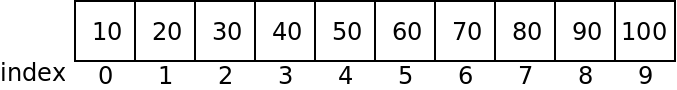Arrays

Introduction

So far we have looked at programs that use scalar variables: ones that can only store one value at a time. There is a lot we can do with these variables, but some things require arrays.

An array is a variable that can hold multiple different values at the same time. They can be used for storing larger sets of information such as:

• The phone numbers of multiple friends.
• The set of prime numbers less than 100.
• The prices of multiple items for sale.

Creating Arrays in Java

To declare an array in Java, we use the following syntax:

<type> <name>[] = new <type>[<size>];
For example, to declare an array of 100 strings called "names":

String names = new String;

Indexing Arrays

An array is accessed by indexing it. This is done with placing the desired index withing square brackets after the array name:

public class Arrays {

public static void main(String args[]) {
String names[] = new String;

names = "Allen";
names = "Bob";
names = "Claire";
names = "Donald";
names = "Ellen";

System.out.println("The second person is " + names);
}
}

Example: Simple Statistics

Given the following code:

import java.util.Random;

class Stats {

// some constants "final" means cannot be changed
public static final int SIZE = 25;
public static final int MAX = 100;

public static void main(String args[]) {
// create the array
int array[] = new int[SIZE];

// fill it up with random numbers
Random generator = new Random();
for (int i = 0; i < SIZE; i++) {
array[i] = generator.nextInt(MAX);
}

}
}

How could we find the smallest, largest, and average value in the array?

Initializing Arrays

If we know ahead of time what values we want in an array, we can initialize it directly using the following syntax:

int numbers[] = new int[] {10, 20, 30, 40, 50, 60, 70, 80, 90, 100};

Note that the size of the array is not specified here. The compiler will figure out that the array needs 10 elements.

Array Storage

Notice that array indices start at zero and go through the size - 1. A common mistake is an "off by one" error in which we mistake the bounds of an array.

Java stores arrays contiguously in memory. The following array:

int numbers [] = {10, 20, 30, 40, 50, 60, 70, 80, 90, 100};

Will be stored as follows:If we access too far to the left, or too far to the right of an array, what happens?

class Fail {
public static void main(String args[]) {
// create the array
int array[] = new int[] {1, 2, 3};

System.out.println("Iterm 3 is: " + array);
}
}

You can find an arrays length for any array with the ".length" member:

class Length {

public static void main(String args[]) {
// create the array
int array[] = new int[] {1, 2, 3};

System.out.println("The length of array is: " + array.length);
}
}

Note that the length minus one is the last element of an array!

Passing Arrays to Functions

The following example passes an array to a function:

class Passing {
public static void printArray(int data[]) {
System.out.print("[");
for (int i= 0; i < data.length; i++) {
System.out.print(data[i] + ", ");
}
System.out.println("\b\b]");
}

public static void main(String args[]) {
// create the array
int array[] = new int[] {1, 2, 3};
printArray(array);
}
}

When passing an array, the size is not included in the parameter. Just empty brackets.

Functions may also return arrays:

import java.util.Random;

class Return {

public static void printArray(int data[]) {
System.out.print("[");
for (int i= 0; i < data.length; i++) {
System.out.print(data[i] + ", ");
}
System.out.println("\b\b]");
}

public static int[] getRandomData(int size, int max) {
int data[] = new int[size];
Random generator = new Random();
for (int i = 0; i < size; i++) {
data[i] = generator.nextInt(max);
}
return data;
}

public static void main(String args[]) {
printArray(getRandomData(10, 10));
}
}

When passing an array to a function, the address of the array in memory is passed. This means changes to the array take effect on the array that is passed in:

import java.util.Random;

class Effects {

public static void printArray(int data[]) {
System.out.print("[");
for (int i= 0; i < data.length; i++) {
System.out.print(data[i] + ", ");
}
System.out.println("\b\b]");
}

public static void clearArray(int data[]) {
for (int i= 0; i < data.length; i++) {
data[i] = 0;
}
}

public static int[] getRandomData(int size, int max) {
int data[] = new int[size];
Random generator = new Random();
for (int i = 0; i < size; i++) {
data[i] = generator.nextInt(max);
}
return data;
}

public static void main(String args[]) {
int array[] = getRandomData(10, 10);
printArray(array);
clearArray(array);
printArray(array);
}
}

Strings as Arrays

String variables in Java can actually be accessed somewhat like arrays. Instead of brackets, we can use the ".charAt" function:

class Strings {

public static void main(String args[]) {
String name = "Ian Finlayson";
for (int i = 0; i < name.length(); i++) {
System.out.println(name.charAt(i));
}
}
}

Note that the characters in a String cannot be changed, however!